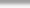## ksh and ksh93: 0: not found [No such file or directory]

When assigning values to an associative array in KSH, it's important to note the significance of the \$ value:

# typeset -A ABCD; \$(( ABCD[“moi”]=0 )); \$(( ABCD[“moi”]++ )); \$(( ABCD[“moi”]++ )); \$(( ABCD[“moi”]++ )); print \${ABCD[“moi”]};
3
#

The solution to the above, is of couse to use this logic (dropping the \$ in front of the (( … )) statements):

# typeset -A ABCD; (( ABCD[“moi”]=0 )); (( ABCD[“moi”]++ )); (( ABCD[“moi”]++ )); (( ABCD[“moi”]++ )); print \${ABCD[“moi”]};
3
#

Cheers,
TK